Open in App
Not now

# How to join tensors in PyTorch?

• Last Updated : 28 Feb, 2022

In this article, we are going to see how to join two or more tensors in PyTorch.

We can join tensors in PyTorch using torch.cat() and torch.stack() functions. Both the function help us to join the tensors but torch.cat() is basically used to concatenate the given sequence of tensors in the given dimension. whereas the torch.stack() function allows us to stack the tensors and we can join two or more tensors in different dimensions such as -1 dimension and 0 dimensions,

torch.cat() function: Cat() in PyTorch is used for concatenating two or more tensors in the same dimension.

Syntax: torch.cat ( (tens_1, tens_2, — , tens_n), dim=0, *, out=None)

torch.stack() function: This function also concatenates a sequence of tensors but over a new dimension, here also tensors should be of the same size.

Syntax: torch.stack ( (tens_1, tens_2, — , tens_n), dim=0, *, out=None)

Example 1:

The following program is to concatenate a sequence of tensors using torch.cat() function.

## Python3

 `# import torch library ` `import` `torch ` ` `  `# define tensors ` `tens_1 ``=` `torch.Tensor([[``11``, ``12``, ``13``], [``14``, ``15``, ``16``]]) ` `tens_2 ``=` `torch.Tensor([[``17``, ``18``, ``19``], [``20``, ``21``, ``22``]]) ` ` `  `# print first tensors ` `print``(``"tens_1 \n"``, tens_1) ` ` `  `# print second tensor ` `print``(``"tens_2 \n"``, tens_2) ` ` `  `# call torch,cat() function ` `# join tensor in -1 dimension ` `tens ``=` `torch.cat((tens_1, tens_2), ``-``1``) ` `print``(``"join tensors in the -1 dimension \n"``, tens) ` ` `  `# join tensor in 0 dimension ` `tens ``=` `torch.cat((tens_1, tens_2), ``0``) ` `print``(``"join tensors in the 0 dimension \n"``, tens) `

Output: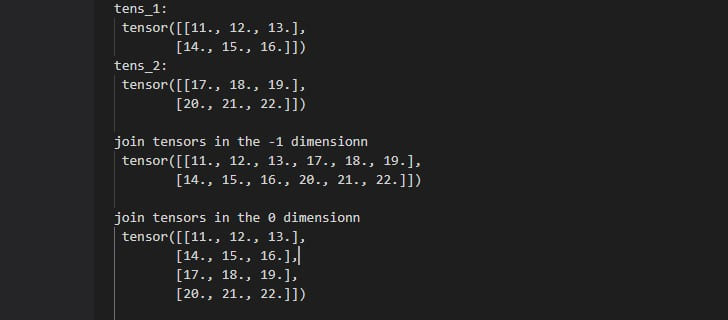Example 2:

The following program is to concatenate a sequence of tensors using torch.stack() function.

## Python3

 `# import torch library ` `import` `torch ` ` `  `# define tensors ` `tens_1 ``=` `torch.Tensor([[``10``,``20``,``30``],[``40``,``50``,``60``]]) ` `tens_2 ``=` `torch.Tensor([[``70``,``80``,``90``],[``100``,``110``,``120``]]) ` ` `  `# print first tensors ` `print``(``"tens_1 \n"``, tens_1) ` ` `  `# print second tensor ` `print``(``"tens_2 \n"``, tens_2) ` ` `  `# call torch,cat() function ` `# join tensor in -1 dimension ` `tens ``=` `torch.stack((tens_1, tens_2), ``-``1``) ` `print``(``"join tensors in the -1 dimension \n"``, tens) ` ` `  `# join tensor in 0 dimension ` `tens ``=` `torch.stack((tens_1, tens_2), ``0``) ` `print``(``"join tensors in the 0 dimension \n"``, tens) `

Output: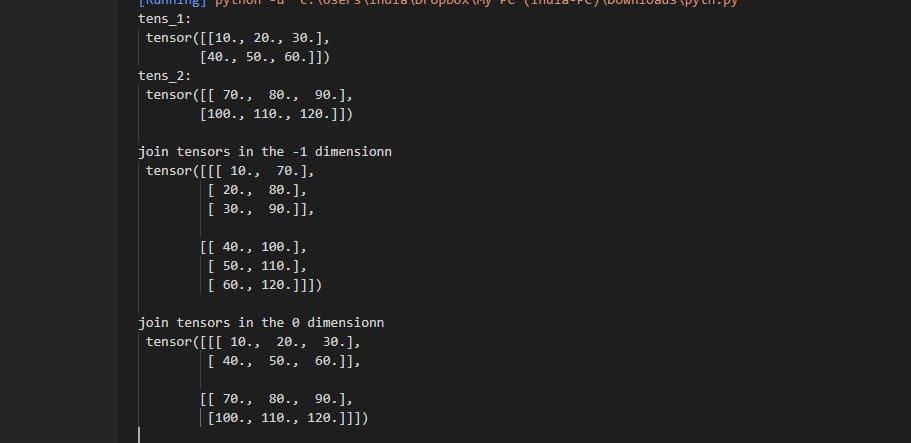Example 3:

The following program is for 2D tensors to be joined (stacked) to create a 3D tensor.

## Python3

 `# import required library ` `import` `torch ` ` `  `# define some tensors ` `tens_1 ``=` `torch.Tensor([[``1``, ``2``], [``3``, ``4``]]) ` `tens_2 ``=` `torch.Tensor([[``5``, ``6``], [``7``, ``8``]]) ` `tens_3 ``=` `torch.Tensor([[``9``, ``10``], [``11``, ``12``]]) ` ` `  `# display tensors ` `print``(``"\n First Tensor :\n"``, tens_1) ` `print``(``"\n Second Tensor :\n"``, tens_2) ` `print``(``"\n Third Tensor :\n"``, tens_3) ` ` `  `# Join (stacked) tensors in -1 dimension ` `tens ``=` `torch.stack((tens_1, tens_2, tens_3), ``-``1``) ` `print``(``"\n tensors in -1 dimension \n"``, tens) ` ` `  `# Join (stacked) tensors in 0 dimension ` `tens ``=` `torch.stack((tens_1, tens_2, tens_3), ``0``) ` `print``(``"\n tensors in 0 dimension \n"``, tens) `

Output: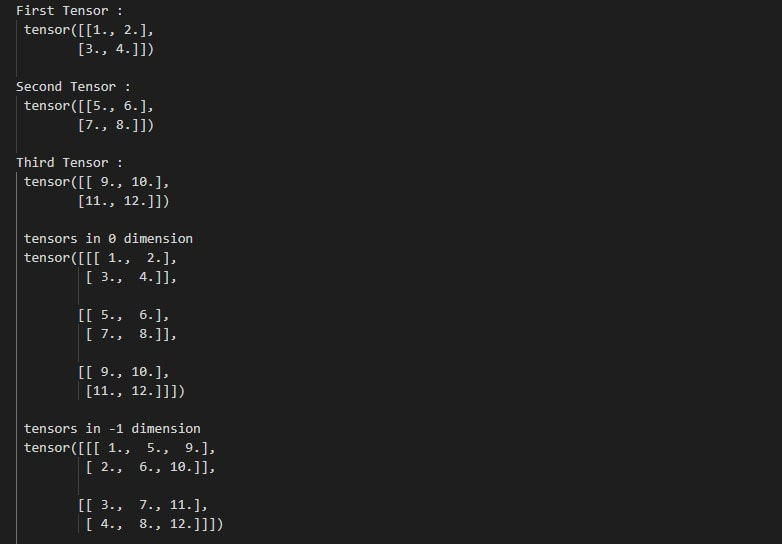Example 4:

The following program is to know how 2D tensors are concatenated along 0 and -1 dimensions. Concatenating in 0 dimension increases the number of rows.

## Python3

 `# import required library ` `import` `torch ` ` `  `# define some tensors ` `tens_1 ``=` `torch.Tensor([[``1``, ``2``], [``3``, ``4``]]) ` `tens_2 ``=` `torch.Tensor([[``5``, ``6``], [``7``, ``8``]]) ` `tens_3 ``=` `torch.Tensor([[``9``, ``10``], [``11``, ``12``]]) ` ` `  `# display tensors ` `print``(``"First Tensor :\n"``, tens_1) ` `print``(``"\nSecond Tensor :\n"``, tens_2) ` `print``(``"\nThird Tensor :\n"``, tens_3) ` ` `  `# join tensors in the 0 dimension ` `tens ``=` `torch.cat((tens_1, tens_2, tens_3), ``0``) ` `print``(``"\n join tensors in the 0 dimension \n"``, tens) ` ` `  `# join tensors in the -1 dimension ` `tens ``=` `torch.cat((tens_1, tens_2, tens_3), ``-``1``) ` `print``(``"\n join tensors in the -1 dimension \n"``, tens) `

Output: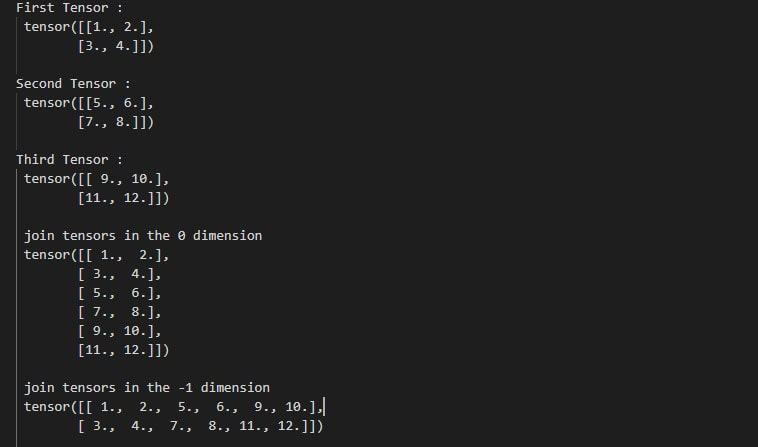Example 5:

The following program is to know how 1D tensors are stacked and the final tensor is a 2D tensor.

## Python3

 `# import required library ` `import` `torch ` ` `  `# define some tensors ` `tens_1 ``=` `torch.Tensor([``1``, ``2``, ``3``]) ` `tens_2 ``=` `torch.Tensor([``4``, ``5``, ``6``]) ` `tens_3 ``=` `torch.Tensor([``7``, ``8``, ``9``]) ` ` `  `# display tensors ` `print``(``"First Tensor :\n"``, tens_1) ` `print``(``"\nSecond Tensor :\n"``, tens_2) ` `print``(``"\nThird Tensor :\n"``, tens_3) ` ` `  `# join tensors in the 0 dimension ` `tens ``=` `torch.stack((tens_1, tens_2, tens_3), ``0``) ` `print``(``"\n join tensors in the 0 dimension \n"``, tens) ` ` `  `# join tensors in the -1 dimension ` `tens ``=` `torch.stack((tens_1, tens_2, tens_3), ``-``1``) ` `print``(``"\n join tensors in the -1 dimension \n"``, tens) `

Output: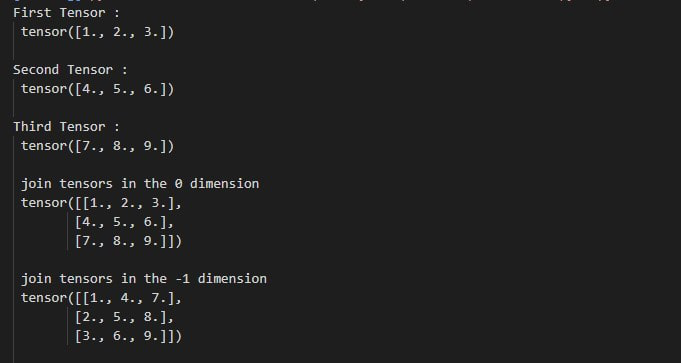My Personal Notes arrow_drop_up
Related Articles